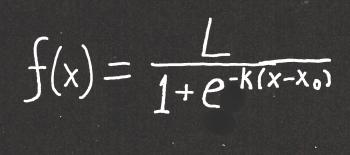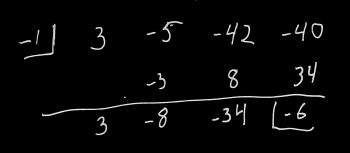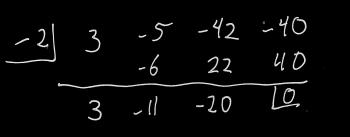Games
Problems
Go Pro!

Category results for 'math'.

William from Arizona asks, "Myself and a fellow math teacher of 20+ years each got into a discussion about extraneous solutions for a particular problem. The problem is this: 1/(x-a) = x/(x-a) The provided answer to this problem is that x = a is always an extraneous solution. However, when you solve it using the LCD method to multiply both sides, the x = a solution candidate does not present itself. (x-a) * 1/(x-a) = x/(x-a) *(x-a) 1 = x cancelling x-a The only candidate after cancelling is x = 1. The counter argument was that you solve by cross multiplying and setting equal getting: x - a = x(x - a) x - a = x^2 - ax 0 = x^2 - ax - x - a 0 = x^2 - (a+1)x - a Using quadratic formula: x = a or x = 1 therefore, x = a is extraneous. Using the cross multiply method, I run into x = a and need to call it extraneous. Using LCD multiply to both sides, it doesn't present as a candidate. So, is it true to say x = a is always an extraneous solution? Thank you for considering"

Hi William, thanks for asking. This is an interesting question. An extraneous solution is generally defined as "a solution to a transformed equation which is not a solution of the original equation." So, for example, if you square both sides of an equation, you've transformed it, and therefore you've introduced the possibility of solutions that might not be solutions to the original equation.

In this case, the transformation that produces an extraneous solution is cross multiplying, which removes the (x - a) from the denominator (and therefore including x = a in the domain).

So an extraneous solution is a solution that arises because of a transformation. If the transformations you performed did not result in a new equation that has an expanded domain, then it doesn't produce extraneous solutions. In other words, no, I don't think you have an extraneous solution using the first method.

The phrasing here is odd; if x = 1 was an extraneous solution, you wouldn't say "x = 1 is always an extraneous solution." I suspect that what they're trying to say is, "it doesn't matter what the value of 'a' is; x = a will never be a solution." So I don't think they were trying to suggest that there's no way to avoid x = a as an extraneous solution, but rather that if it does arise as a solution, it is always extraneous, regardless of the value of 'a'.

As one last note, I'd like to point out that you can avoid using the quadratic formula in your second method:

(x - a) = x(x - a)
0 = x(x - a) - (x - a)  //subtract (x - a) from both sides
0 = (x - a)(x - 1) //use the distributive property to rewrite the previous equation
x =a or x = 1

Wesley asks, "I have been reading your answers to the corona virus questions and came across the term logistic curve. I am interested in the math behind them, and do not see how you can make a logistic curve unless one of two things. One, you take the inverse of an odd degree polynomial or two somehow you can incorporate the last y-value to influence the next x-value. I have come across this problem before and I would like to know if there is an alternate solution that can make a logistic curve an actual function of y in terms of x. If there isn't, thank you anyways for your time and effort."

That's a great question, Wesley. I like your thinking regarding odd degree polynomials. Odd degree polynomials do have an inflection point similar to a logistic curve. The problem with polynomial equations is that they don't have asymptotes. An asymptote is a straight line that the graph approaches without ever touching. A simple example of a graph with asymptotes is xy = 1. That graph gets closer and closer to the x and y axes, but never touches it.

A logistic curve has two horizontal asymptotes - one at the base of the curve, and one at the top. So a polynomial function (even an odd one) won't do the trick. What we need is an exponential function. Here's what you're looking for:This is a messy looking function, but hopefully I can talk you through the concepts fairly simply. First, just in case you (or another reader) doesn't know what "e" is - it's the natural logarithm, which is approximately 2.71828. L, k, and x0 are also constants. The are set values for any particular problem or real-world application.

A "normal" exponential function might look something like this: f(x) = ekx. k controls how quickly the function "blows up" and x is the independent variable. Since we're thinking about virus spread, the independent variable is time - perhaps the number of days that have passed.

The logistic curve has elements of an exponential curve. It's interesting that the "e" is in the denominator, and also that it has a negative exponent - those two features sort of "cancel" each other out, because a negative exponent indicates taking the reciprocal.  But it's the other stuff in the denominator with the e that makes this function's behavior interesting.

First, if x < x0, then the exponent of e is a positive number, and the denominator will be growing larger the further x is from x0. This results in a large denominator, which results in the value of the fraction approaching 0.

On the other hand, if x > x0, then e has a negative exponent, which means it is getting closer and closer to 0. This means the denominator is getting closer and closer to 1, and the function value is getting closer and closer to L.

This gives us our two asymptotes: y = 0 and y = L. Thus, 0 < f(x) < L.

And the point x = x0 is the point at which the function switches from blowing up to leveling off.

TO SUMMARIZE: in the logistic curve, k determines the rate at which the function grows, L represents the maximum value, and x0  represents the point in time at which the graph has its inflection point. In terms of a viral infection, L is the number of people who will be infected, and x0 is the time at which the number of new infections begins decreasing.

Wesley writes, "Is there a better way of factoring polynomials other than guess and check? For example: factoring 21x- 2x2 + 4x - 1, you probably will have to guess and check what goes in the parentheses. It could be (3x - something)(7x + something)(x - something)..., or, it could be something entirely different. Aside from the limited equations like difference of squares, or sum/difference of cubes, I would like to know if it is possible to not have to guess and check. If you do not know of a solution, thank you anyways for your time."

Hi Wesley, In addition to the rules that you mentioned, you can find a few more rules here, including sum/difference of odd powers, sum of even powers, grouping, and something I like to call aggressive grouping.

If none of those help, another tool that might help you out is something called the Rational Root Theorem. The rational root theorem says that if a rational zero to a polynomial exists, then written in simplest form p/q, p is a factor of the constant, and q is a constant of the highest order coefficient.

How does this help in factoring? Well, if p/q is a zero of the polynomial, then (qx - p) is a factor.

It's important to note that the statement above has a big "if" in it. It's possible that no rational roots exist. But if they do exist, this rule helps us out in our trial-and-error search by eliminating possibilities.

Let's take an example: 3x3 - 5x2 - 42x - 40 = 0.

The rational root theorem tells us that if this has rational roots, they are of the form p/q, where p is a factor of 40, and q is a factor of 3. Now, this still gives us a LOT of possibilities to consider, because p could be 1, 2, 4, 5, 8, 10, 20, or 40 (plus the opposites of all of those), and q could be either 1 or 3 (or their opposites).

So let's start with something that's not a fraction: Let's try 1, and see what happens. I'm going to do synthetic division on this. If you don't know how synthetic division works, you'll want to take a look at this explanation: How to do synthetic division.This left us with a remainder of -6, and we're looking for something that gives us a remainder of 0. Let's try 2:Ah ha! That did it. That means we know that -2 is a root, or (x - (-2)) is a factor.

The next bit of good news is that we can use the values in our synthetic division to reduce the polynomial by the factor we just found: our polynomial can be rewritten as (x + 2)(3x2 - 11x - 20) (note the numbers at the bottom of our synthetic division - they are the coefficients of the reduced polynomial). To find our next factors we can use the techniques that we're familiar with on the factor 3x2 - 11x - 20, or we can continue doing synthetic division.

Of course, I cheated; I created a polynomial which I knew could be factored, but there's no guarantee that a given polynomial is factorable over real numbers, let alone rational numbers! Your polynomial, for example, has only one real root, and that one real root is not rational. If you had tried the rational root theorem on this, you would have tried 1, 1/3, 1/7, 1/21, and the opposites of all of those, only to find that none of them work. The good news is that because of the rational root theorem, you KNOW that you're done looking for rational roots.

So there are a few more tools for your arsenal; I hope something here was helpful!

Here's a fun question regarding an upcoming snowstorm:

"4 to 8 plus 4 to 8 equals 8 to 16, right?"

This is in reference to a prediction that tomorrow there will be 4 - 8 inches of snow, and then tomorrow night there will be 4 - 8 inches of snow. The questioner is suggesting that this means there will be a total of 8 - 16 inches of snow.

It seems reasonable, right? Except we're all pretty sure we're not going to see anywhere near 16 inches of snow in the next 48 hours. So why can't we just add these two ranges together?Meterologists have a LOT of unknowns they have to deal with (and I'm not a meteorologist, so I'm not going to pretend I know even a tenth of the variables they deal with). But what I do know is that one of the broad uncertainties is when a storm will pass through. If you look at the chart here (taken from an actual hourly forecast on the National Weather Service website), you'll see that they don't actually know when the storm will start; the snowfall bars begin (with low probability) at 6:00 AM. It's not until noon that they're saying with confidence, "We'll have snowfall by now."

So hypothetically, if the snowstorm starts at 6:00 AM, and goes all day, maybe we'll get 8 inches of snow during the day. But if that happens, the storm will be mostly over by evening, and maybe we'll get 4 inches overnight.

On the other hand, if the storm doesn't start until noon, maybe we'll get 4 inches during the day. But then the storm will last longer into the night, and maybe we'll get 8 inches overnight.

What I've just described is a scenario in which we get a maximum total of 12 inches. But if we were to break it down by how much falls during the day and how much falls at night, we'd have to say "4 - 8 during the day" and "4 - 8 during the night" - even though we feel confident that the storm won't give us more than 12 inches total.

What we're seeing is that a portion of the snowfall could come during the day, and it could come at night. Since we don't know when, it gets listed in both time periods.

Watch the forecasts and see how they line up from storm to storm, and you'll see what I mean: if the daytime forecast is accurate, the overnight forecast will likely be overkill. And vice-versa. Of course, we don't always notice this, because as the storm gets closer, the meteorologists are able to refine their models better.

Then we just whine that the meteorologists have been "hyping up the storms." :D

As I mentioned before, there are undoubtedly many many more variables that I'm not considering, but my purpose here was to show wny you can't just sum the ranges to get a total.

Maizi from Norwich asks, "Four positive whole numbers add up to 84 One of the numbers is a multiple of 17 The other 3 numbers are equal. What are they?"

Hi Maizi, as is my custom with math problems, I'm not going to answer your question; instead I'm going to invent a similar problem and show you the technique for solving it. This will allow you the satisfaction of solving this particular problem on your own. So here is my problem:

Five positive whole numbers add up to 97, and one of them is a multiple of 19. The other four numbers are all equal.  What are they?

First, we consider that if we add together four equal numbers, the result must be a multiple of four. For example, 7 + 7 + 7 + 7 = 28, or 11 + 11 + 11 + 11 = 44.

Algebraically, we'd say that n + n + n + n = 4n.

So this means that if we subtract the first number (the one that's a multiple of 19) from 97, we will have a result that is a multiple of 4.

What could the first number be? 19, 38, 57, 76, or 95. Any other value is larger than the sum of 7, which is not possible if all the integers are non-negative.

Based on this, we can find all possible sums of the other four numbers, by subtracting each of the numbers above from 97:

97 - 19 = 78
97 - 38 = 59
97 - 57 = 40
97 - 76 = 21
97 - 95 = 2

Remember what I said earlier? The other four numbers must add to a multiple of 4. The only one of those sums above that is a multiple of 4 is 40. Therefore, we conclude that the first number is 57, and the other four numbers are each 10 (because 40 / 4 = 10).

I hope that's helpful; good luck with your version of the problem!

Older posts# Blogs on This SiteReviews and book lists - books we love!The site administrator fields questions from visitors.Like us on Facebook to get updates about new resources